Well-structured OP Malhotra Class 9 Maths Solutions Chapter 8 Triangles Ex 8(A) facilitate a deeper understanding of mathematical principles.

## S Chand Class 9 ICSE Maths Solutions Chapter 8 Triangles Ex 8(A)

Question 1.
In the figure, AB and CD bisect each other at K. Prove that AC = BD.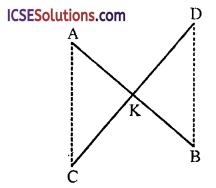Solution:
Given: Two line segments AB and CD bisect each other at K. AC and BD are joined.To prove : AC = BD
Proof: In ∆AKC and ∆BKD
AK = BK (given)
CK = DK (given)
∠AKC = ∠BKD (vertically opposite angles)
∆AKC ≅ ∆BKD (SAS axiom)
AC = BD (c.p.c.t.)
Hence proved.

Question 2.
In the figure, the sides BA and CA have been produced such that BA = AD and CA = AE. Prove that DE || BC.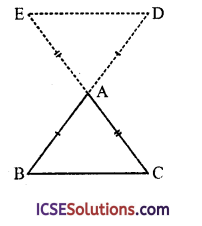Solution:
Given : In ∆ABC, sides BA and CA are produced such that BA = AD and CA = AE. ED is joined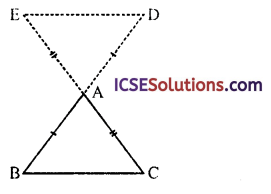To prove : DE || BC
Proof: In ∆BAC and ∆DAE
BA = DA (given)
CA = EA (given)
∠BAC = ∠DAE (vertically opposite angles)
∴ ∆BAC = ∆DAE (SAS axiom)
But these are alternate angles
∴ DE || BC
Hence proved.

Question 3.
In the figure, ABCD is a rectangle; P is the; mid-point of AB, Q and R are points in AD and BC respectively such that AQ = BR. Prove that PQ = PR.Solution:
Given : ABCD is a rectangle. P is the mid point of AB. Q and R are points in AD and BC respectively such that AQ = BR PO and PR are joined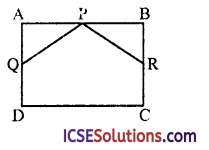To prove : PQ = PR
Proof: In ∆PAQ and ∆PBR
PA = PB (∵ P is mid point of AB)
∠A = ∠B (each 90°)
AQ = BR (given)
∴ ∆PAQ ≅ ∆PBR (SAS axiom)
PQ = PR (c.p.c.t.)
Hence proved.Question 4.
In the figure, OA = OB, OC = OD, ∠AOB = ∠COD, prove that AC = BD.Solution:
Given : In the figure OA = OB
OC = OD, ∠AOB = ∠COD
To prove : AC = BD
Proof: ∵ ∠AOB = ∠COD
∴ ∠AOC + ∠COB = ∠DOB + ∠COB
⇒ ∠AOC = ∠BOD
In ∆OAC and ∆ODB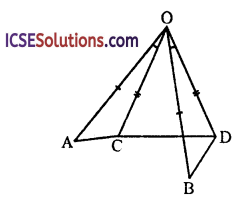OA = OB (given)
OC = OD (given)
and ∠AOC = ∠BOD (proved)
∴ ∆OAC ≅ ∆ODB (SAS axiom)
∴ AC = BD (c.p.c.t)
Hence Proved.

Question 5.
In figure, prove that ∆s OAM and OBN are congruent and hence prove that AM = BN.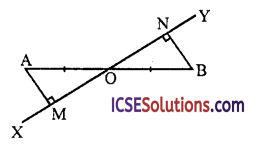Solution:
Given : In the figure
OA = OB
AM ⊥ XY and BN ⊥ XYTo prove :
(i) ∆OAM s ∆OBN
(ii) AM ≅ BN
Proof:
(i) In ∆OAM and ∆OBN
OA = OB (given)
∠M = ∠N (each 90°)
and ∠AOM = ∠BON
(vertically opposite angles)
∴ ∆OAM ≅ ∆OBN (AAS axiom)

(ii) Hence AM = BN (c.p.c.t.)

Question 6.
In Figure, ∠XYZ is bisected by YP. L is any point in YP and MLN is perpendicular to YP. Prove that LM = LN.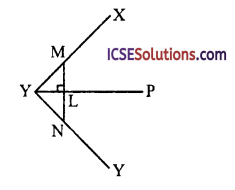Solution:
Given : In the figure,
YP is the bisector of ∠XYZ
L is any point on YB and MLN is perpendicular to YP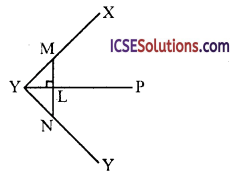To prove : LM = LN
Proof: In ∠YML and ∆YNL
YL = YL (common)
∠YLM = ∠YLN (each 90°)
∠MYL = ∠NYL (∵ YP is the bisector of ∠XYZ)
∴ ∆YML ≅ ∆YNL (ASA axiom)
∴ LM = LN (c.p.c.t.)
Hence proved.Question 7.
In figure, X is any point within a square ABCD. On AX a square AXYZ is described. Prove that BX = DZ.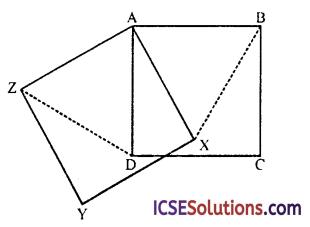Solution:
Given : In the figure,
ABCD and AXYZ are squares where X is any point within the square ABCD
BX and DZ are joined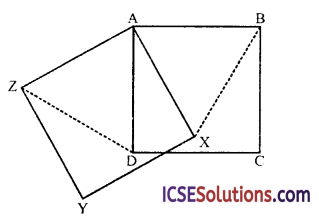To prove : BX = DZ Proof: In ∆ABX and ∆ADZ,
AB = AD (sides of the square ABCD)
AX = AZ (sides of the square AXYZ)
∠B AX = ∠DAZ (each = 90° – ∠D AX)
∴ ∆ABX = ∆ADZ (SAS axiom)
∴ BX = DZ (c.p.c.t.)
Hence proved.

Question 8.
In figure, prove that AP bisects ∠BAC.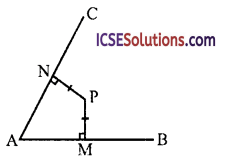Solution:
Given : In the figure, P is any point inside the ∠BAC
PM ⊥ AB and PN ⊥ A are drawn and PM = PNTo prove : AP is the bisector of ∠BAC
Proof : In right ∆PAM and ∆PAN
Side PM = PN (given)
Hyp PA = PA (common)
∴ ∆PAM ≅ ∆PAN (RHS axiom)
∴ ∠PAM – ∠PAN (c.p.c.t.)
∴ PA is the bisector of ∠B AC
Hence proved.

Question 9.
In figure, if PR – PS, PT = PQ, ∠PTS = ∠PQR = 90°, prove that RQ – ST and RT = SQ.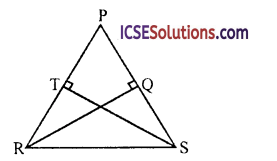Solution:
Given : In the figure, PR = PS, PT = PQ, ∠PQR = 90° and ∠PTS = 90°To prove :
(i) RQ = ST
(ii) RT = SQ
Proof:
(i) In right ∆PQR and ∆PTS
Side PQ = PT (given)
Hyp. PR = PS (given)
∴ ∆PQR ≅ ∆PTS (RHS axiom)
∴ RQ = ST (c.p.c.t)

(ii) ∵ PR = PS
and PT = PQ
Subtracting we get,
PR – PT = PS – PQ
⇒ RT = SQ
Hence proved.

Question 10.
In figure, PQRS is a square. Arc AB is drawn with centre P and any radius less than PR, cutting SR at A and RQ at B. Prove that AS = BQ.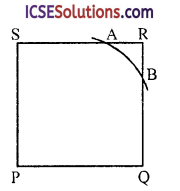Solution:
Given : In square PQRS an arc AB is drawn with centre P and a radius less then PR which intersects SR at A and RQ at B
To prove : AS = BQ
Construction : Join PA and PBProof : In right ∆PQB and ∆PSA
Side PQ = PS (sides of a square)
Hyp. PB = PA (radii of the same arc AB)
∴ ∆PQB = ∆PSA (RHS axiom)
∴ BQ = AS (c.p.c.t.)
or AS = BQ
Hence proved.Question 11.
In figure, if LM = MN, QM = MR and ∠MLQ = ∠MNR = 90°, prove that PQ = PR.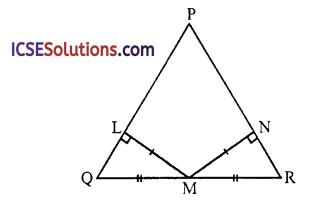Solution:
Given : In the figure, ∆PQR in which
LM = MN, QM = MR and
∠MLQ = ∠MNR = 90°To prove : PQ = PR
Proof: In right ∆QLM and ∆RNM
Side LM = MN (given)
Hyp. QM = MR
∴ ∆QLM = ∆RNM (RHS axiom)
∴ ∠Q = ∠R (c.p.c.t.)
Now in APQR
∵ ∠Q = ∠R (proved)
∴ PR = PQ (sides opposite equal angles)
or PQ = PR
Hence proved.

Question 12.
In figure, ∆ABC is right-angled at B. ∆CDE and ∆CGF are squares. Prove that
(i) ∆BCD ≅ ∆ACG;
(ii) AG = BD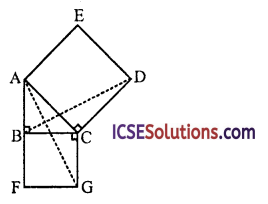Solution:
Given : ∆ABC is a right angled, right angle at B. ACDE and BCGF are squares on the sides AC and BC respectively. AG and BD are joined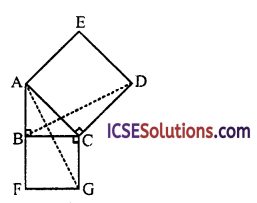To prove :
(i) ∆BCD = ∆ACG
(ii) AG = BD
Proof: ∵ ∠ACD = ∠BCG (each 90°)
∠ACD + ∠ACB = ∠ACB + ∠BCG
⇒ ∠BDC = ∠ACG
Now in ∆BCD and ∆ACG
BC = CG (sides of the same square)
∠BCD = ∠ACG (proved)
CD = AC (side of the same square)
∴ ∆BCD ≅ ∆ACG (SAS axiom)
∴ BD = AG (c.p.c.t.)
or AG = BD
Hence proved.

Question 13.
Prove that the medians of an equilateral triangle are equal.
Solution:
Given : ∆ABC is an equilateral triangle in which AD, BE and CF are its medians.To prove : AD = BE = CF
Proof: In ∆EBC and ∆FBC
BC = BC (common)
EC = FB
(Half of equal sides of equilateral triangle)
∠C = ∠B (each 90°)
∴ ∆EBC ≅ ∆FBC (SAS axiom)
∴ BE = CF … (i)
Similarly we can prove that
∴ BE = AD …. (ii)
From (i) and (ii),
Hence proved.

Question 14.
In figure, AC = DE, ∠ACB =∠EDF and BD = CF. Prove that AB = EF.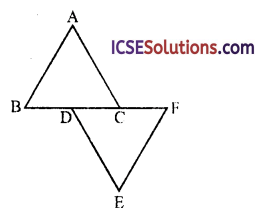Solution:
Given : In the figure,
AC = DE, ∠ACB =∠EDF
and BD = FC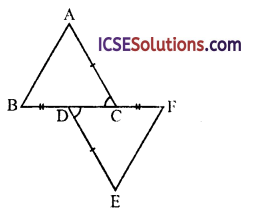To prove : AB = EF
Proof:
∵ BD = CF
BD + DC = DC + CF
⇒ BC = DF
Now in ∆ABC and ∆DEF
AC = DE (given)
BC = DF (proved)
∠ACB = ∠EDF (given)
∴ ∆ABC ≅ ∆DEF (SAS axiom)
∴ AB = EF (c.p.c.t.)
Hence proved.

Question 15.
In figure, AC = AE, AB = AD and ∠BAD =∠EAC. Prove that BC = DE.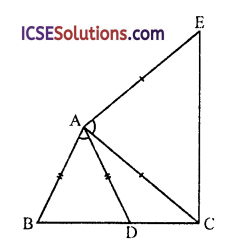Solution:
In the figure,
To prove : BC = DE
Construction : Join DEProof:
∴ ∠BAD + ∠DAC = ∠DAC + ∠EAC
AC = AE (given)
∠BAC = ∠DAE (proved)
∴ ∆ABC ≅ ∆ADE (SAS axiom)
∴ BC = DE (c.p.c.t.)
Hence proved.Question 16.
In figure, PS is a median and QL and RM are perpendiculars drawn from Q and R respectively on PS and PS produced. Prove that QL = RM.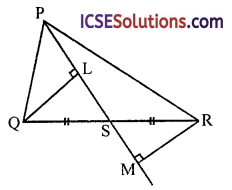Solution:
In ∆PQR, PS is the median of side QR
QL and RM are perpendiculars on the medianTo prove : QL = RM
Proof:
In ∆QLS and ∆RMS
QS = SR (S is mid point of QR)
∠QSL = ∠RSM (vertically opposite angles)
∠QLS = ∠RMS (each 90°)
∆QLS ≅ ∆RMS (AAS axiom)
∴ QL = RM (c.p.c.t.)
Hence proved.

Question 17.
ABCD is a parallelogram. The sides AB, AD are produced to E, F so that AB = BE and AD = DF. Prove that the triangles BEC and DCF are congruent. (SC)
Solution:
Given : ABCD is a parallelogram, sides AB and AD are produced to E and F respectively such that AB = BE and AD = DF
To prove : ∆BEC ≅ ∆DCF
Construction : Join EC and FC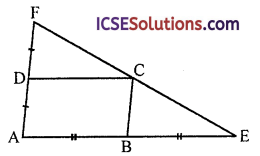Proof: In ||gm ABCD,
∠DAB = ∠FDC = ∠CBE (corresponding angles)
Now in ABEC and ∆DCF
BC = DF (each = AD)
BE = DC (each = AB)
and ∠CBE = ∠FDC (proved)
∴ ∆BEC ≅ ∆DCF (SAS axiom)
Hence proved.

Question 18.
In figure, QX and RX are the bisectors of the angles Q and R respectively of the triangle PQR. If XS ⊥ QR and XT ⊥ PQ, prove that,
(i) ∆XTQ ≅ ∆XSQ;
(ii) PX bisects the angle P.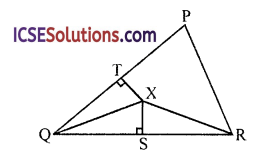Solution:
Given : In the figure, QX and RX are the bisectors of the angles Q and R respectively of the triangle PQR and XS ⊥ QR and XT ⊥ PQ
To prove :
(i) ∆XTZ ≅ ∆XSQ
(ii) PX bisects the angle P
Construction : Join PX and draw XV ⊥ PRProof:
In ∆XQS and ∆XTQ,
XQ = XQ (common)
∠S = ∠T (each 90°)
∠XQS = ∠XQT (∵ XQ is bisector of ∠Q)
∴ ∆XQS ≅ ∆XTQ (AAS)
or ∆XTQ = ∆XSQ
∴ XS = XT (c.p.c.t.) … (i)
Similarly we can prove that
∆XSR ≅ ∆XVR
∴ XS = XV … (ii)
From (i) and (ii) XT = XV
Now in ∆ right XTP and ∆XYP
Hyp. XP = XP (common)
Side XT = XV (proved)
∴ ∆XTP ≅ ∆XYP (RHS axiom)
∴ ∠XPT = ∠XPV (c.p.c.t.)
∴ PX bisects ∠P
Hence proved.

Question 19.
Find the values ofx and y in the figures given below, using congruency of triangles.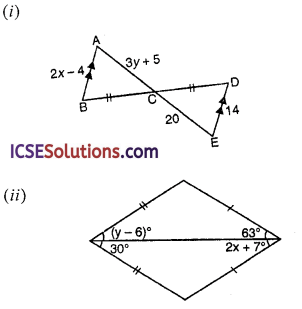Solution:
(i) In the figure (i) AB || DE and BC = CD
In ∆ABC and ∆DEC,
BC = CD (given)
∠ACB = ∠ECD (vertically opposite angles)
∠B = ∠D (alternate angles)
∴ ∆ABC ≅ ∆DEC (ASA axiom)
∴ AB = DE (c.p.c.t.)
⇒ 2x – 4 = 14 ⇒ 2x = 14 + 4 = 18
⇒ x = $$\frac { 18 }{ 2 }$$ = 9
∴ x = 9
and AC = CE (c.p.c.t.)
⇒ 3y + 5 = 20 ⇒ 3y = 20 – 5 = 15
⇒ y = $$\frac { 15 }{ 3 }$$ = 5
Hence x = 9 and y = 5

(ii) In figure (ii)
AB = AD, BC = DC
∠BAC = (y – 6)°, ∠BCA = 63°
∠CAD = 30° and ∠ACD = (2x + 7)°
⇒ x = $$\frac { 56° }{ 2 }$$ = 28°